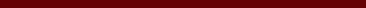### STUDI ALGORITMA IGVNS, ALGORITMA GVNS, DAN ALGORITMA ABC PADA MULTIPLE TRIP VEHICLE ROUTING PROBLEM (MTVRP)

Nurul Faridhatul Aini, Vita Kusumasari, Desi Rahmadani

#### Abstract

The Multiple Trip Vehicle Routing Problem (MTVRP) is one of the VRP variants with vehicle capacity constraints, and the limited number of vehicles allows each vehicle to distribute more than one route so as to minimize the number of vehicles used. The algorithm used in this study is the Improved General Variable Neighborhood Search (IGVNS) algorithm. The IGVNS algorithm is a hybrid between the GVNS algorithm and the VNS algorithm. The calculation results of the IGVNS algorithm will be compared with the GVNS algorithm and the ABC algorithm. The main stages of the IGVNS and GVNS algorithms are initial solution formation, perturbation, and solution improvement. The main stages of the ABC algorithm are initialization, solution improvement and optimization. Based on manual calculations using 8 points, the ABC algorithm produces a distance of 86 km and a service time of 1.47 hours, the GVNS algorithm produces a distance of 80 km and a service time of 1.37 hours, the IGVNS algorithm produces a distance of 79 km and service time is 1.35 hours. Based on one example of the ABC algorithm, the calculation solution using the IGVNS algorithm shows more optimal results. Based on the results of parameter testing, the  parameter affects the calculation results, that is the greater the  value, the more optimal the resulting solution. While the  parameter does not affect the calculation results because it shows constant results in two successive iterations carried out.

#### Full Text:

FULL PDF

DOI: http://dx.doi.org/10.17977/um055v2i22021p26-31

### Refbacks

• There are currently no refbacks.